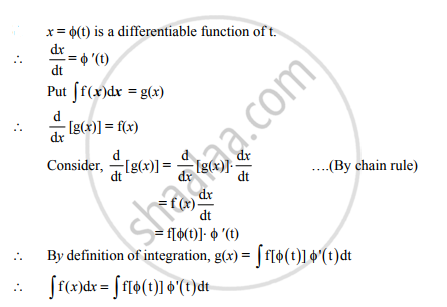# If x = Φ(t) differentiable function of ‘ t ' then prove that ∫f(x) dx=∫f[Φ(t)] Φ'(t)dt - Mathematics and Statistics

If x = Φ(t) differentiable function of ‘ t ' then prove that int f(x) dx=intf[phi(t)]phi'(t)dt

#### SolutionConcept: General and Particular Solutions of a Differential Equation
Is there an error in this question or solution?